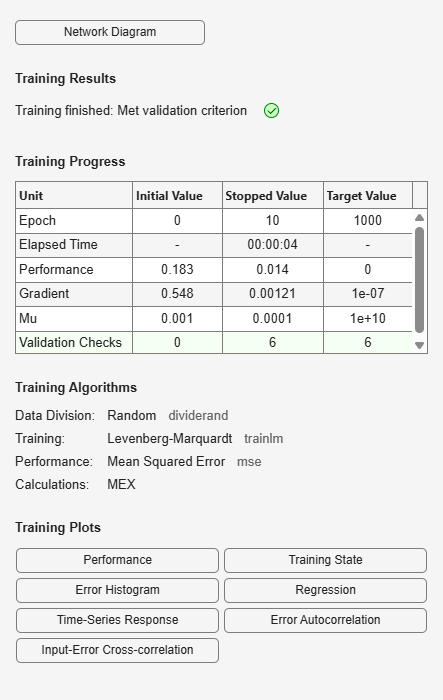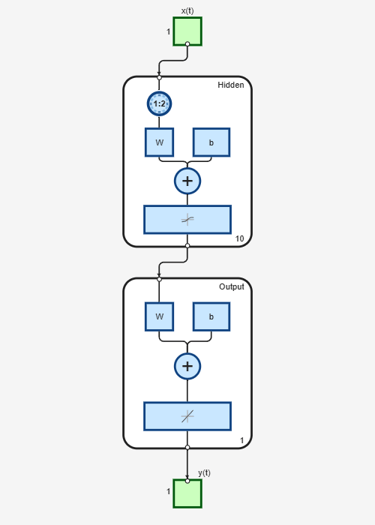Documentation

timedelaynet

Time delay neural network

Syntax

timedelaynet(inputDelays,hiddenSizes,trainFcn)

Description

Time delay networks are similar to feedforward networks, except that the input weight has a tap delay line associated with it. This allows the network to have a finite dynamic response to time series input data. This network is also similar to the distributed delay neural network (distdelaynet), which has delays on the layer weights in addition to the input weight.

timedelaynet(inputDelays,hiddenSizes,trainFcn) takes these arguments,

 inputDelays Row vector of increasing 0 or positive delays (default = 1:2) hiddenSizes Row vector of one or more hidden layer sizes (default = 10) trainFcn Training function (default = 'trainlm')

and returns a time delay neural network.

Examples

collapse all

Partition the training set. Use Xnew to do prediction in closed loop mode later.

[X,T] = simpleseries_dataset;
Xnew = X(81:100);
X = X(1:80);
T = T(1:80);

Train a time delay network, and simulate it on the first 80 observations.

net = timedelaynet(1:2,10);
[Xs,Xi,Ai,Ts] = preparets(net,X,T);
net = train(net,Xs,Ts,Xi,Ai);
view(net)Calculate the network performance.

[Y,Xf,Af] = net(Xs,Xi,Ai);
perf = perform(net,Ts,Y);

Run the prediction for 20 timesteps ahead in closed loop mode.

[netc,Xic,Aic] = closeloop(net,Xf,Af);
view(netc)y2 = netc(Xnew,Xic,Aic);